Foxtable(狐表)用户栏目专家坐堂 → 选中行后筛选

共有492人关注过本帖树形打印复制链接

# 主题：选中行后筛选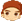11楼 | 信息 | 搜索 | 邮箱 | 主页 | UC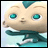加好友发短信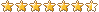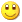Post By：2020/6/3 9:39:00 [只看该作者]

 下载信息  [文件大小：   下载次数： ]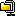点击浏览该文件:选中筛选(1).zip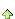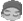yangwenghd
12楼 | 信息 | 搜索 | 邮箱 | 主页 | UC加好友发短信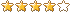Post By：2020/6/3 9:46:00 [只看该作者]

 谢谢老师，感谢 感谢yangwenghd
13楼 | 信息 | 搜索 | 邮箱 | 主页 | UC加好友发短信Post By：2020/6/5 21:51:00 [只看该作者]

 老师，如果要清除之前选中的记忆应该怎么写呀，比如我现在筛选，选中，然后后面切换后会就自动勾选到开启项目时候被选中的行，可以是点击按钮，然后就重新开始记忆吗？感谢 感谢 If e.Button = MouseButtons.Right Then  '右键点击显示Tables("窗口1_Table1").ClearCheckedRowsEnd If14楼 | 信息 | 搜索 | 邮箱 | 主页 | UC加好友发短信Post By：2020/6/6 8:55:00 [只看该作者]

 清空按钮lst.clearyangwenghd
15楼 | 信息 | 搜索 | 邮箱 | 主页 | UC加好友发短信Post By：2020/6/6 20:35:00 [只看该作者]

 老师，我可以把这两段代码融合到一起吗？就是切换的时候不要全部都显示出来，是筛选后的，感谢 感谢     Dim t As Table = e.Form.Controls("Table2").Table    For Each r As Row In t.GetCheckedRows        If lst.contains(r("_Identify")) = False            lst.Add(r("_Identify"))        End If    Next        t.ApplyFilter = not t.ApplyFilter        For Each r As Row In t.Rows        If lst.Contains(r("_Identify")) Then            r.Checked  = True        End If    NextTables("采订录入窗口_Table2").Filter = "企业链号 Like '%" & e.Form.Controls("ComboBox4").Text & "%'"  '  筛选出行\16楼 | 信息 | 搜索 | 邮箱 | 主页 | UC加好友发短信Post By：2020/6/7 20:42:00 [只看该作者]

 Dim t As Table = e.Form.Controls("Table2").Table    For Each r As Row In t.GetCheckedRows        If lst.contains(r("_Identify")) = False            lst.Add(r("_Identify"))        End If    Next        t.Filter = "企业链号 Like '%" & e.Form.Controls("ComboBox4").Text & "%'"        For Each r As Row In t.Rows        If lst.Contains(r("_Identify")) Then            r.Checked  = True        End If    Nextyangwenghd
17楼 | 信息 | 搜索 | 邮箱 | 主页 | UC加好友发短信Post By：2020/6/12 14:58:00 [只看该作者]

 老师 ，能在帮忙看看这段吗？就是如果没有选中（一行都没有选中）就会弹出下面的错误，可以是如果一行都没有选中就不显示吗？ 感谢 感谢 .NET Framework 版本：2.0.50727.9151Foxtable 版本：2018.10.9.1错误所在事件：详细错误信息：语法错误: IN 关键字后面必须是由逗号分隔的非空表达式列表，并且必须用括号括起来。Dim t As Table = e.Form.Controls("Table1").TableFor Each r As Row In t.GetCheckedRows    If lst.contains(r("_Identify")) = False        lst.Add(r("_Identify"))    End IfNextDim s As String = ""For Each r As Row In Tables("采订录入窗口_Table1").GetCheckedRows    s =s & "," & r("_Identify")Nexts = s.Trim(",")t.Filter = "[_Identify] in (" & s & ")"For Each r As Row In t.Rows    If lst.Contains(r("_Identify")) Then        r.Checked  = True    End IfNext18楼 | 信息 | 搜索 | 邮箱 | 主页 | UC加好友发短信Post By：2020/6/12 15:09:00 [只看该作者]

 Dim s As String = ""For Each r As Row In Tables("采订录入窗口_Table1").GetCheckedRows    s =s & "," & r("_Identify")Nextif s = "" then returnyangwenghd
19楼 | 信息 | 搜索 | 邮箱 | 主页 | UC加好友发短信Post By：2020/6/12 15:36:00 [只看该作者]

 下载信息  [文件大小：   下载次数： ]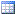点击浏览该文件:选中筛选.table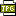此主题相关图片如下：qq截图20200612153415.jpg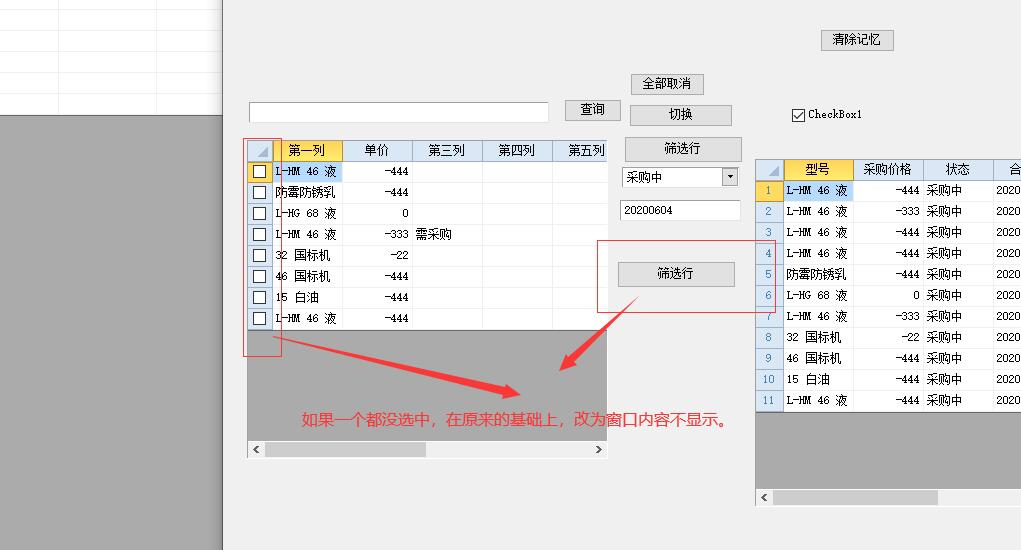20楼 | 信息 | 搜索 | 邮箱 | 主页 | UC加好友发短信Post By：2020/6/12 15:55:00 [只看该作者]

 Dim s As String = ""For Each r As Row In Tables("采订录入窗口_Table1").GetCheckedRows    s =s & "," & r("_Identify")Nextif s = "" then t.Filter = “false”returnend if总数 23 上一页 1 2 3 下一页